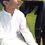# Intresting combination & probability problem

I am a DOTA 2 player and I came across this intriguing problem which I do not know how to solve. In this game 1 match is played between two teams, each consisting of 5 players. Each player has to pick a hero/character from a pool of total 107 heroes, i.e 10 heroes picked. Moreover both team's captain bans 5 heroes for other team to pick. i.e 10 heroes banned.

Now, in a tournament of 16 teams, played on a knockout format with each contest between two teams consisting of 3 matches (best of 3 winner) and grand final of 5 matches. What is the probability that atleast X (lets say 5) heroes will not be picked or banned in whole tournament.Note by Musabbir Hussain
7 years, 2 months ago

This discussion board is a place to discuss our Daily Challenges and the math and science related to those challenges. Explanations are more than just a solution — they should explain the steps and thinking strategies that you used to obtain the solution. Comments should further the discussion of math and science.

When posting on Brilliant:

• Use the emojis to react to an explanation, whether you're congratulating a job well done , or just really confused .
• Ask specific questions about the challenge or the steps in somebody's explanation. Well-posed questions can add a lot to the discussion, but posting "I don't understand!" doesn't help anyone.
• Try to contribute something new to the discussion, whether it is an extension, generalization or other idea related to the challenge.

MarkdownAppears as
*italics* or _italics_ italics
**bold** or __bold__ bold
- bulleted- list
• bulleted
• list
1. numbered2. list
1. numbered
2. list
Note: you must add a full line of space before and after lists for them to show up correctly
paragraph 1paragraph 2

paragraph 1

paragraph 2

[example link](https://brilliant.org)example link
> This is a quote
This is a quote
    # I indented these lines
# 4 spaces, and now they show
# up as a code block.

print "hello world"
# I indented these lines
# 4 spaces, and now they show
# up as a code block.

print "hello world"
MathAppears as
Remember to wrap math in $$ ... $$ or $ ... $ to ensure proper formatting.
2 \times 3 $2 \times 3$
2^{34} $2^{34}$
a_{i-1} $a_{i-1}$
\frac{2}{3} $\frac{2}{3}$
\sqrt{2} $\sqrt{2}$
\sum_{i=1}^3 $\sum_{i=1}^3$
\sin \theta $\sin \theta$
\boxed{123} $\boxed{123}$

Sort by:

I don't know of any simple formula for this one, but here's what I came up with:

In the whole tournament, there will be $(8+4+2) \cdot 3 + 1 \cdot 5 = 47$ matches. That translates to $47 \cdot 20 = 940$ hero-selections (either for picking or banning, that isn't our concern).

If you don't want $y$ players to be selected in the whole tournament, then, effectively in every match you have $\displaystyle N_y = 107 - y$ heroes. So, in each match, you can have $\displaystyle {N_y \choose 20}$ selections. And since there are $940$ matches, totally there are $\displaystyle {N_y \choose 20}^{940}$ possible selections.

Thus, the probability of $y$ heroes being completely neglected in the whole tournament is $\displaystyle P(y) = \dfrac{ {N_y \choose 20}^{940} } { {107 \choose 20}^{940} }$.

So, the probability that at least $X$ are totally neglected in the whole tournament is:

$\displaystyle 1 - \sum_{0 \le y \le {X-1} } P(y)$

- 7 years, 2 months ago

And since there are $1140$ matches,

Isn't there only 14 matches. Other than that, good solution

- 7 years, 2 months ago

Thank you Parth, But i think ( 8 + 4 + 2 ) x 3 + 1 x 5 = 47...So, there will be 47 matches in total 15 contests..that would mean 47 x 20 = 940 hero-selections. Right?

A good solution though.

- 7 years, 2 months ago

Aah that was so dumb! :D Thanks for pointing it out. Dumb Dumb Dumb! Sometimes its fun to do dumb things though ;) :D Edited.

- 7 years, 2 months ago

No Problem..I also do such dumb things alot :D..! another thing I would like to understand is that why did u put ( 940 20 ) in denominator..Can you please explain the equation in words. I am sorry if that is something too obvious. Sadly, I am much interested but not so good in Probability, Combination & Permutation :-S

- 7 years, 2 months ago

That's another mistake I made! Well, it should be ${107 \choose 20}$ and not ${940 \choose 20}$. That's the total number of possible selections, without any player being neglected.

- 7 years, 2 months ago

One question. Can both teams ban the same hero?

- 7 years, 2 months ago

No. A hero can be picked/banned only once.

- 7 years, 2 months ago